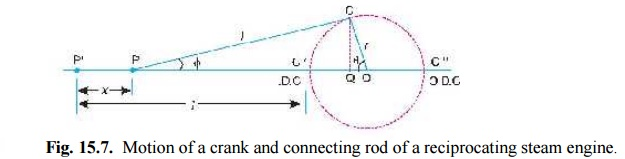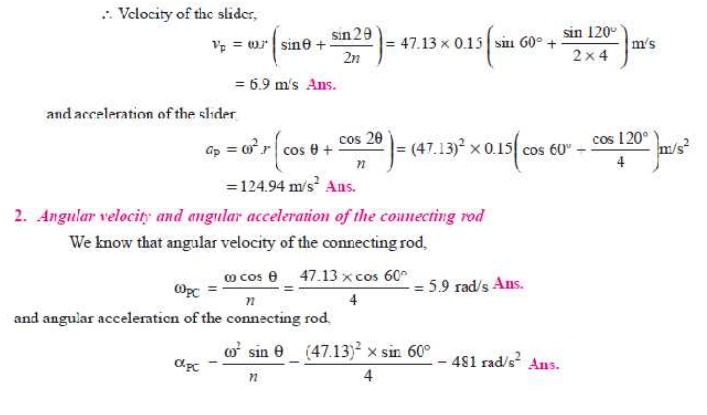Home | | Dynamics of Machines | Approximate Analytical Method for Velocity and Acceleration of the Piston

# Approximate Analytical Method for Velocity and Acceleration of the PistonConsider the motion of a crank and connecting rod of a reciprocating steam engine as shown in Fig. 15.7. Let OC be the crank and PC the connecting rod.

APPROXIMATE ANALYTICAL METHOD FOR VELOCITY AND ACCELERATION OF THE PISTON

Consider the motion of a crank and connecting rod of a reciprocating steam engine as shown in Fig. 15.7. Let OC be the crank and PC the connecting rod. Let the crank rotates with angular velocity of Ď‰ rad/s and the crank turns through an angle Î¸ from the inner dead centre (briefly written as I.D.C). Let x be the displacement of a reciprocating body P from I.D.C. after time t seconds, during which the crank has turned through an angle Î¸ .Fig. 15.7. Motion of a crank and connecting rod of a reciprocating steam engine.

Let     l         =       Length of connecting rod between the centres,

r        =       Radius of crank or crank pin circle,

Ď† =    Inclination of connecting rod to the line of stroke PO, and

n        =       Ratio of length of connecting rod to the radius of crank = l/r.

Velocity of the pistonAcceleration of the piston

Since the acceleration is the rate of change of velocity, therefore acceleration of the piston P,Q.In a slider crank mechanism, the length of the crank and connecting rod are 150 mm and 600 mm respectively. The crank position is 60Â° from inner dead centre. The crank shaft speed is 450 r.p.m. (clockwise). Using analytical method, determine: 1. Velocity and acceleration of the slider, and 2. Angular velocity and angular acceleration of the connecting rod.

Solution. Given : r = 150 mm = 0.15 m ; l = 600 mm = 0.6 m ; Î¸ = 60Â°; N = 400 r.p.m or Ď‰ = Ď€ Ă— 450/60 = 47.13 rad/s

1. Velocity and acceleration of the slider

We know that ratio of the length of connecting rod and crank,

n = l / r = 0.6 / 0.15 = 4Study Material, Lecturing Notes, Assignment, Reference, Wiki description explanation, brief detail
Mechanical : Dynamics of Machines : Force Analysis : Approximate Analytical Method for Velocity and Acceleration of the Piston |